# ROW Function In ExcelArticle byExcelMojo Team## What Is ROW Function In Excel?

The ROW Function in Excel is used to find the row number of a cell reference or a cell range, and not its value. In a large dataset the function quickly finds the row index. The ROW Function in Excel is an inbuilt function, so we can enter it as a formula or insert it from the Function Library, Lookup & Reference group.

For example, the following table contains a requirements list for what row number should get displayed in each cell in the cell range B2:B4.

We will perform the required tasks in cell range B2:B4, using ROW function.

The output is shown above.

• In the first case, display the row number of cell B2, the function does not need any argument.
• Next, display the row number of cell B50. [B50 is the argument].
• In the 3rd scenario, when the argument is a cell range, the ROW function in Excel is the row number of the topmost row in the range (Refer to row 4 in the above table).
###### Key Takeaways
• The ROW function in Excel gives the row number of a specific cell reference. Thus, the function is useful when we have to find a row number in a massive dataset or perform calculations requiring a row number.
• The ROW function accepts one argument, i.e., reference, which is also optional.
• One of the arguments, i.e., reference, in the ROW function can be an empty value, cell reference, or reference to a cell range.
• In some advanced scenarios, we can use the ROW function along with other Excel functions such as MOD, INT, INDEX, MATCH, and HLOOKUP.

### ROW() Excel Formula

The syntax of the ROW formula in Excel is:

The argument of the ROW function is,

• reference: The cell or cell range to find the row number. It is an optional argument.

### How to Use ROW Excel Function?

We can enter the Row Excel formula in 2 ways, namely:

1. Access from the Excel Ribbon.
2. Use in the worksheet manually.

#### Method #1 – Access from the Excel ribbon

Choose an empty cell for the output 🡪 select the “Formulas” tab 🡪 go to the “Function Library” 🡪 click the “Lookup & Reference” drop-down 🡪 select the “Row” function, as shown below.

The Row Excel formula is inserted in the selected cell, and the “Function Arguments” window appears instantly. Enter the Excel cell reference or cell range, and click “OK”.

[Note: If we enter the ROW() formula without providing the reference argument, we will get the cells row number where the formula is entered, as output.]

#### Method #2 – Use in the worksheet manually

1. Select a target cell or cell range for output.
2. Type the formula =ROW(. [Alternatively, type =R or =RO and double-click the ROW() function from the list of Excel suggestions.]
3. Enter the row argument, i.e., the cell reference or the cell range.
4. Press the “Enter” key.

Let us take a basic example to perform the required tasks using ROW in Excel.

In the following table, the data is,

• Columns A, B, and C contain a list of fruits and their order details.
• Column E contains the tasks to be performed.

The steps to perform the tasks using ROW function in Excel are,

1. Select cell F2, enter the formula =ROW() and press the “Enter” key. The result is shown below.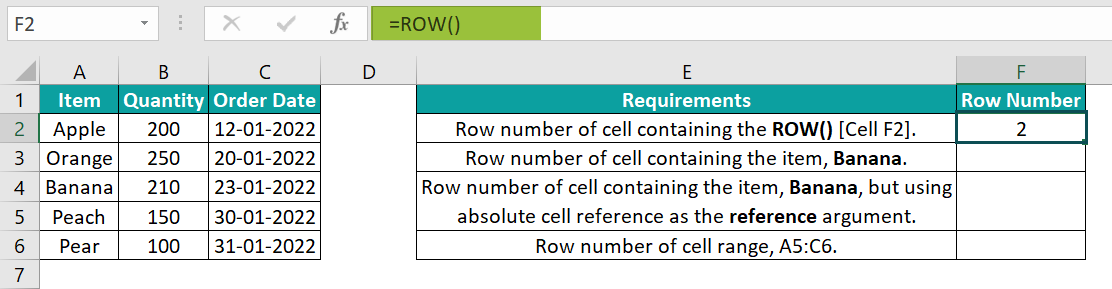2. Next, select cell F3, enter the formula =ROW(A4), and press the “Enter” key. The result is shown below.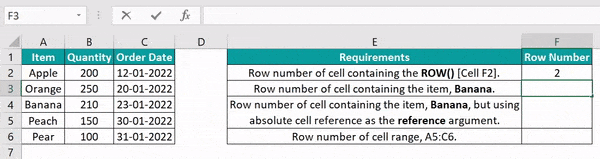[Alternatively, select cell F3 🡪 select the “Formulas” tab 🡪 go to the “Function Library” 🡪 click the “Lookup & Reference” drop-down 🡪 select the “Row” function, to open the “Function Arguments” window, as shown below.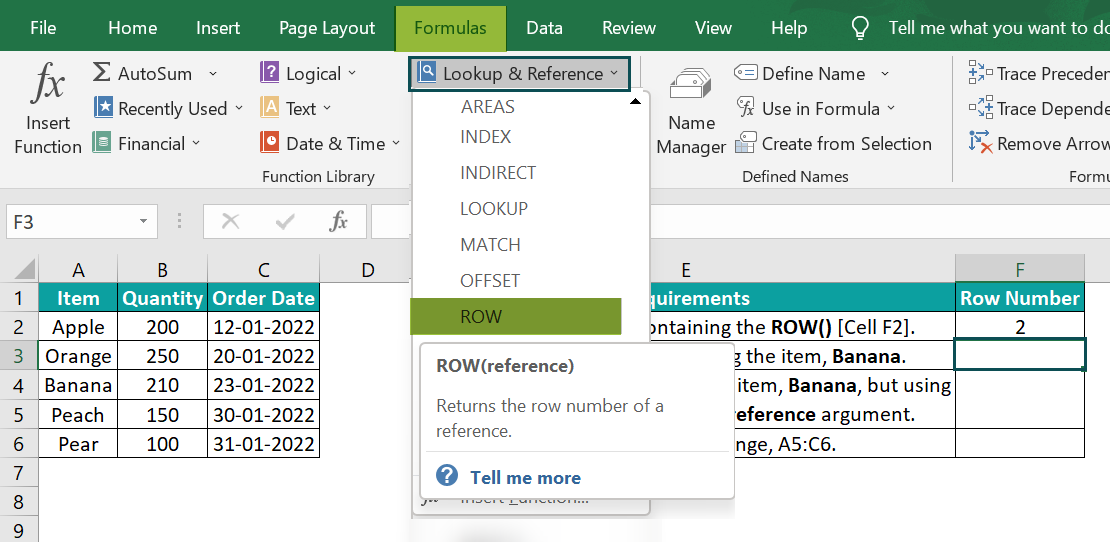The Row Excel formula is inserted in the selected cell, and the “Function Arguments” window appears instantly. Now, enter A4 in the “Reference” field.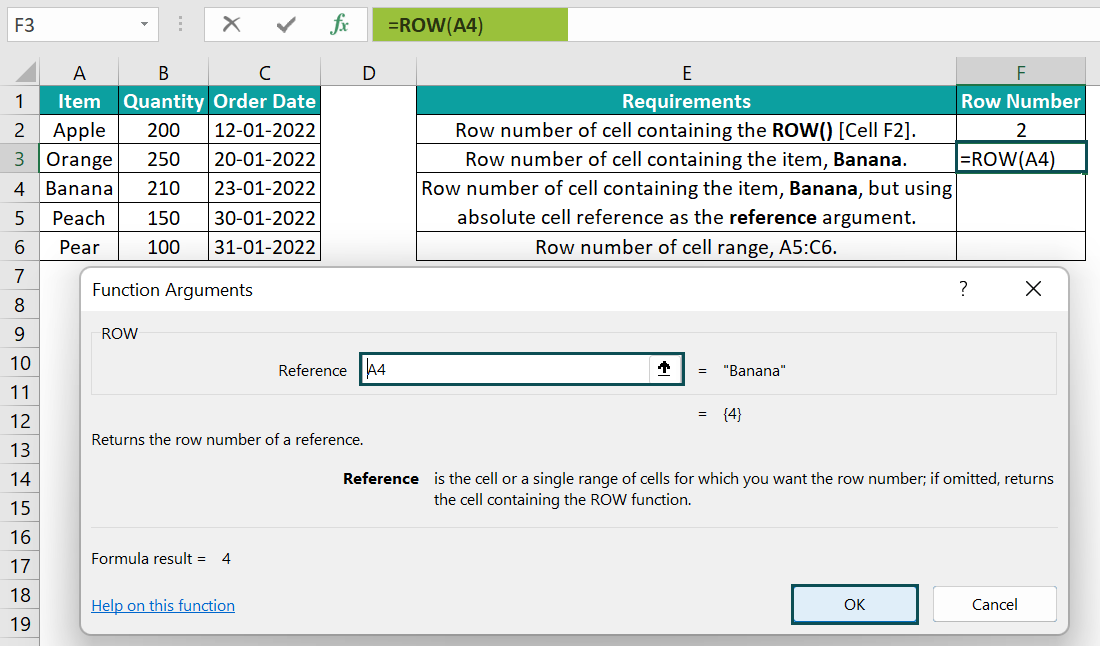Click “OK”. We get row number 4 as the output in cell F3.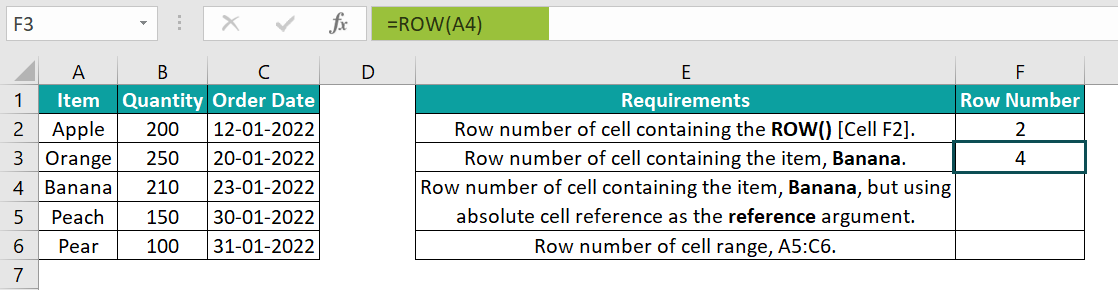3. Now, select cell F4, enter the formula =ROW(\$A\$4), and press the “Enter” key. The result is shown below.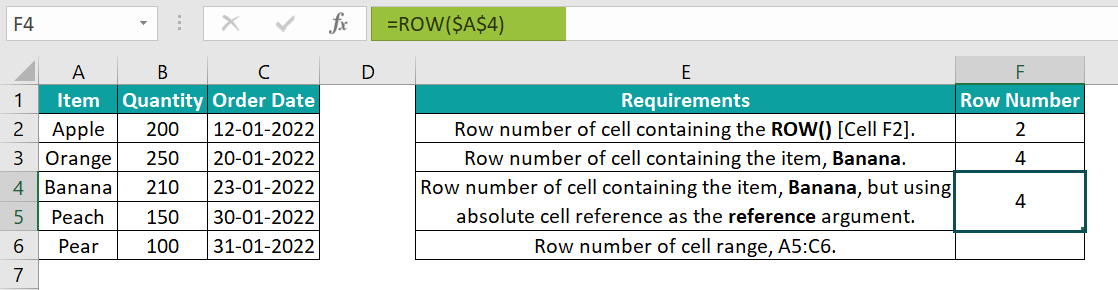4. Finally, select cell F4, enter the formula =ROW(A5:C6), and press the “Enter” key. The result is shown below.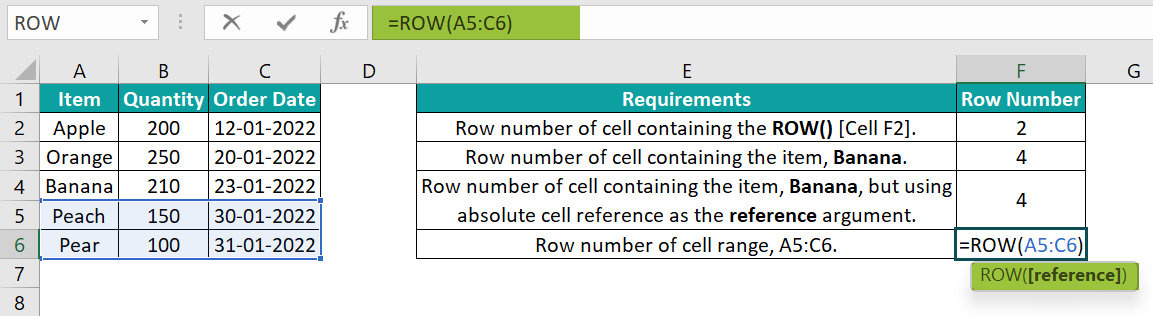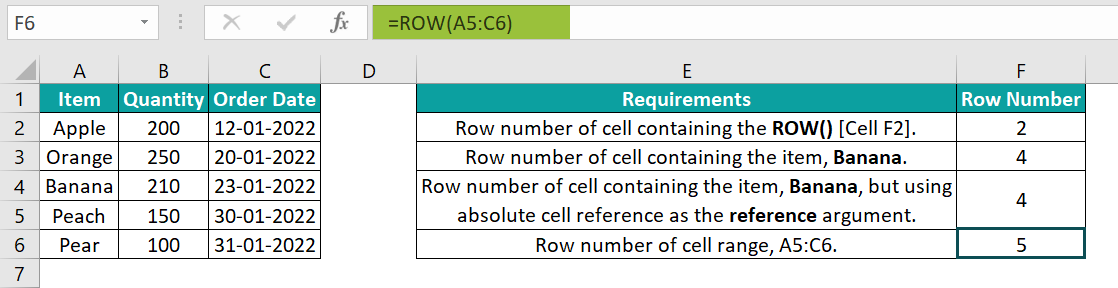In the above example we see various scenarios of the reference argument in the ROW function.

### Examples

Let us understand how to insert ROW function in Excel with some advanced scenarios.

#### Example #1

We will insert ROW function to highlight a set of rows along with the MOD() and INT() functions for the scores of five students in different subjects.

In the following table, the data is,

• Columns A, B, and C contain the Student Names, Subjects, and Scores, respectively.

The steps to use the ROW function in Excel with MOD and INT functions to show the alternating subjects’ rows in a different color are as follows:

1: Select cell range A2:C21 🡪 go to the “Home” tab 🡪 go to the “Styles” group 🡪 click the “Conditional Formatting” drop-down 🡪 select the “New Rule” option, as shown below.

2: The “New Formatting Rule” window pops up.

• Go to the “Select a Rule Type:” group 🡪 choose the “Use a formula to determine which cells to format” option.
• Go to the “Edit the Rule Description:” 🡪 type the formula =MOD(INT((ROW()-2)/5)+1,2) in the “Format values where this formula is true:” field.
• Click the “Format” button.

3: The “Format Cells” window pops up. Go to the “Fill” tab 🡪 go to the “Background Color” group 🡪 choose the desired color, here, Green 🡪 click “OK”. The “Format Cells” window closes.

4: Click “OK” to close the “New Formatting Rule” window.

The output is shown below with the alternating subjects’ rows shaded in Green.

#### Example #2

Let us insert ROW function with the HLOOKUP() function to display the population of a specific state.

The following table contains US states, capitals, and population details.

The procedure to display the population of Connecticut, using the ROW function and HLOOKUP() are follows:

Select the target cell B7, enter the formula =HLOOKUP(B6,B1:K3,ROW(G3),0) and press the “Enter” key. The output is shown below.

In the above example, the ROW function formula returns row number 3 as the output. So, the HLOOKUP() becomes: =HLOOKUP(B6,B1:K3,3,0)

The function then performs a horizontal lookup to return Connecticut’s population as ‘3605597’, as shown above.

#### Example #3

We will use the ROW function in Excel with INDEX() function and MATCH() functions to find the row number with the details of a specific employee.

In the following table, the data is,

• Columns A, B, and C contain the Employee Names, Designation, and Performance Rating, respectively.

The procedure to determine the row number for the employee, Greg Hogan, are as follows:

Select cell F2, enter the formula=ROW(INDEX(A2:C21,MATCH(E2,A2:A21,0),1)), and press the “Enter” key. The output is shown below.

In the above example, MATCH() returns the value 8, as Greg Hogan is the eighth name on the employee list. Then the INDEX() returns the absolute cell reference \$A\$9, based on the array A2:C21, row 8, and column 1. And finally, the ROW() displays the row number 9, based on the cell reference, \$A\$9.

Thus, we can refer to row number 9 to get Greg Hogan’s record.

### Important Things to Note

• The argument in the ROW function in Excel cannot be multiple references.
• A ROW() function, without the argument value or any cell reference, will return the row number of the cell where we entered the ROW function.
• If the supplied argument is not a proper cell reference, such as a reference to a cell or cell range, we get the #NAME? error.
• If the argument of the ROW() function is a cell range, the function will return the topmost row in the particular cell range.

1. Where is the ROW function in Excel?

The ROW function in Excel is in the Formulas tab. Click Formulas 🡪 Lookup & Reference 🡪 ROW to access it.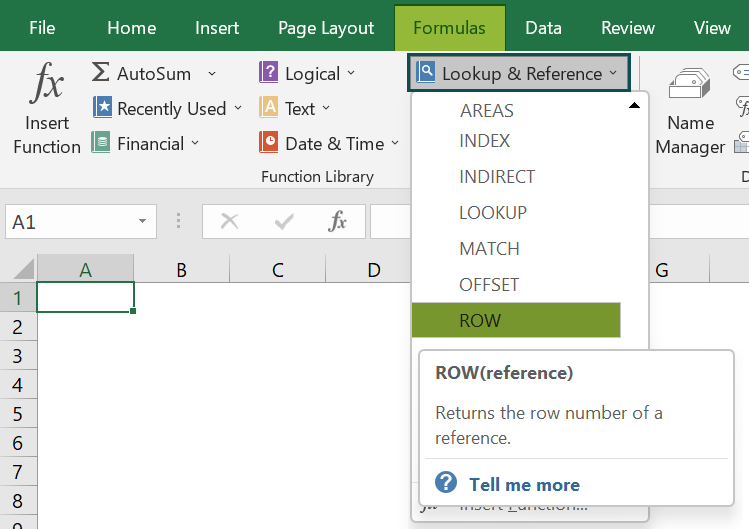2. Why is the ROW function not working in Excel?

The ROW function in Excel might not work due to the following reasons:
● The specified cell or cell range is invalid.
● Multiple references are provided as the argument to the ROW function.
● We provided an argument that is not a proper cell reference.

3. How to execute the ROW function in Excel VBA?

We can execute the ROW function in Excel VBA, using the below steps as shown in the following example.
The following table shows a list of products and their prices.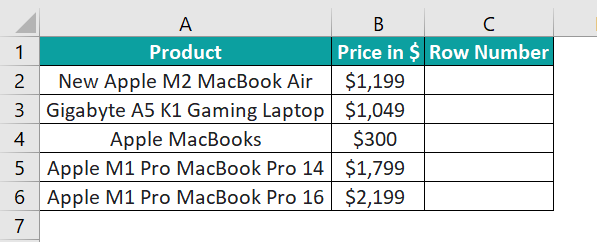The steps to find the row number of each product using the ROW function and the Excel VBA are:
1: In the worksheet with the above table, press the shortcut keys Alt + F11 to open the “VBA Editor”.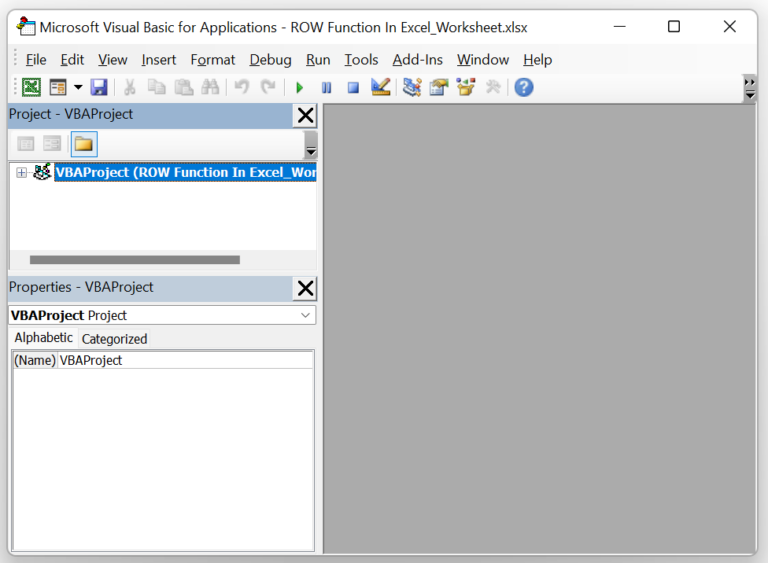2: Click on the required VBAProject in the menu on the left (as depicted in the image above) and select Insert 🡪 Module to open a new module window.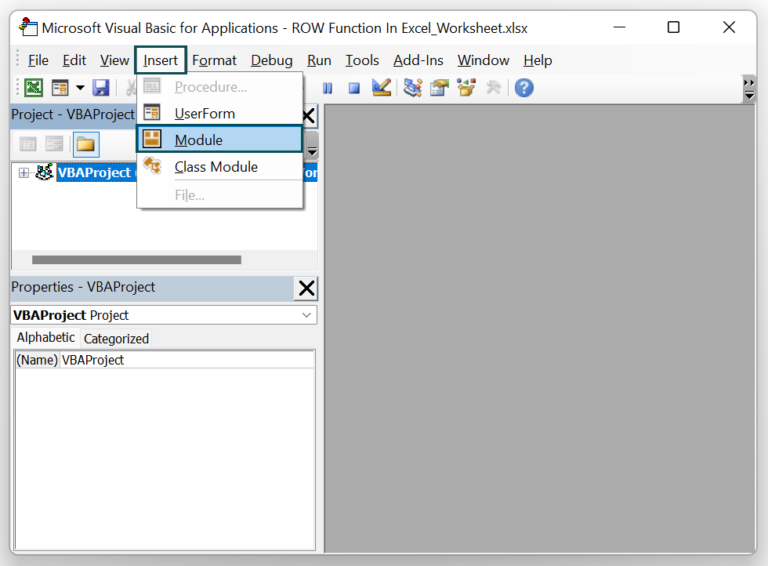3: Once the Module 1 window opens, type the VBA code, shown in the below image, to display the required row numbers in the target cells C2:C6.

Sub Excel_ROW_Fn()
Dim rn As Worksheet
Set rn = Worksheets(“ROW_FAQ”)
rn.Range(“C2”) = rn.Range(“A2”).Row
rn.Range(“C3”) = rn.Range(“A3”).Row
rn.Range(“C4”) = rn.Range(“A4”).Row
rn.Range(“C5”) = rn.Range(“A5”).Row
rn.Range(“C6”) = rn.Range(“A6”).Row
End Sub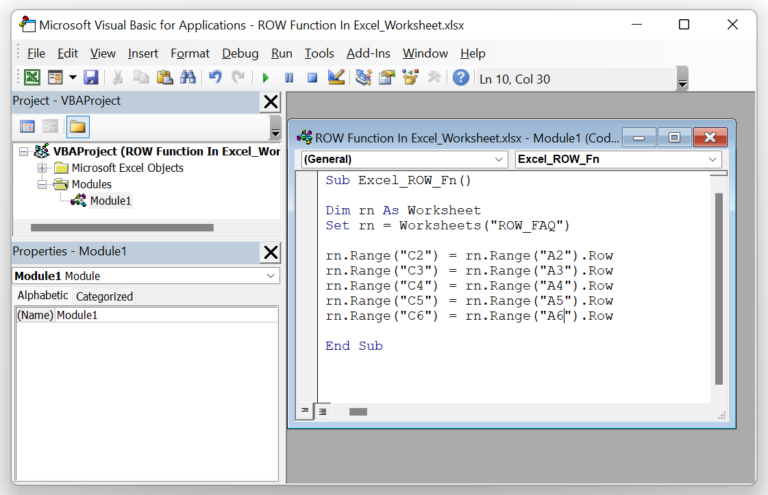4: Next, click the Run Sub/UserForm option in the menu to execute the commands.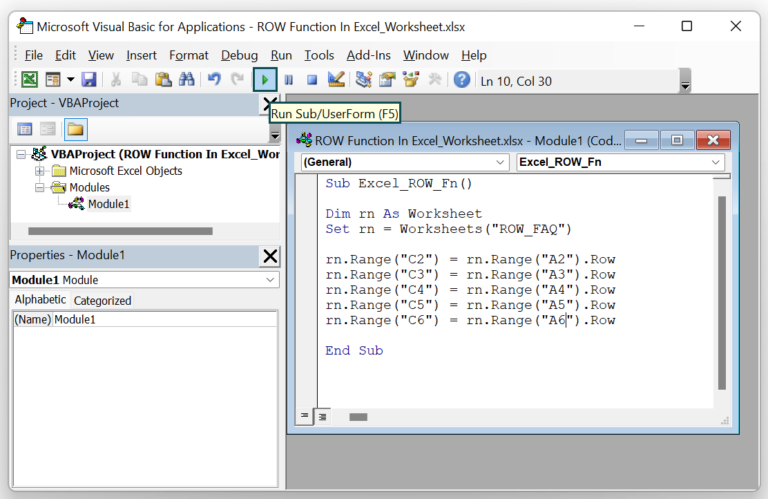5: Finally, open the worksheet to view the output with the row numbers displayed in column C.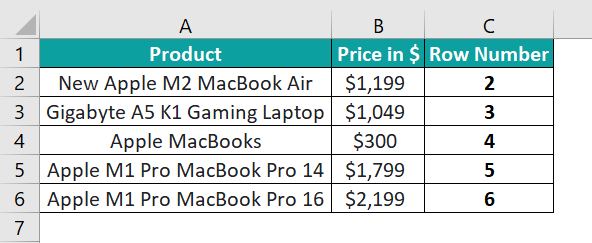Likewise, suppose we have to show the row number of a cell range, say A2:C6, in the target cell C6. Then the VBA code will be as shown in the image below:

Sub Excel_ROW_Fn()
Dim rn As Worksheet
Set rn = Worksheets(“ROW_FAQ”)
rn.Range(“C2”) = rn.Range(“A2”).Row
rn.Range(“C3”) = rn.Range(“A3”).Row
rn.Range(“C4”) = rn.Range(“A4”).Row
rn.Range(“C5”) = rn.Range(“A5”).Row
rn.Range(“C6”) = rn.Range(“A2:C6”).Row
End Sub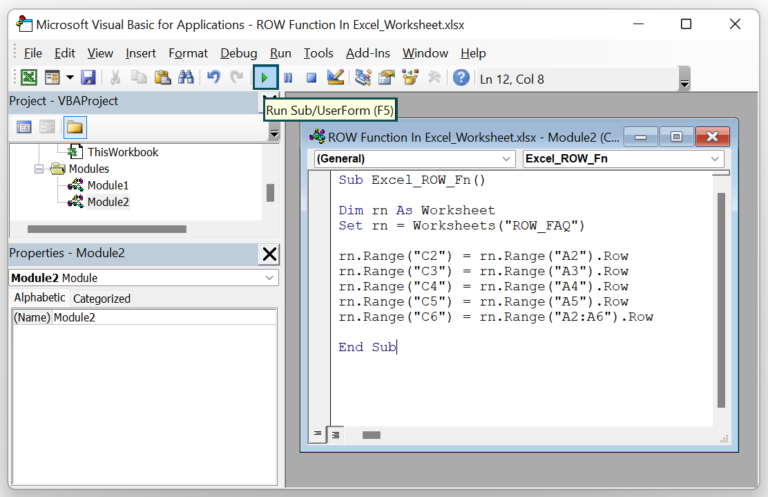And the new output will be: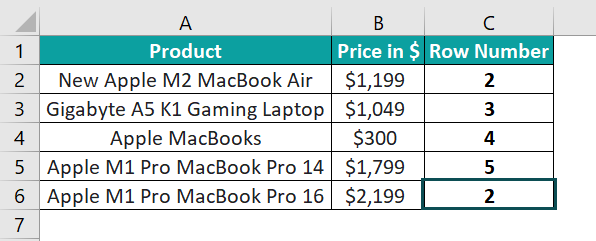Cell C6 shows row number 2, the topmost row in the cell range, A2:C6.

4. What is the difference between the ROW and ROWSfunctionsn in Excel?

The difference between the ROW and ROWS functions in Excel is that the ROW() function gives the row number of the specified cell or cell range reference. On the other hand, the ROWS() function determines the number of rows in the specified reference as a number.